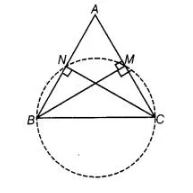# If BM and CN are the perpendiculars`
Question:

If BM and CN are the perpendiculars drawn on the sides AC and AB of the ΔABC, prove that the points B, C, M and N are concyclic.

Solution:

Given In ΔABC, BM ⊥ AC and CN ⊥ AB.

To prove Points B, C, M and N are con-cyclic.

Construction Draw a circle passing through the points B, C, M and N.Proof Suppose, we consider SC as a diameter of the circle. Also, we know that SC subtends a 90° to the circle.

So, the points M and N should be on a circle.

Hence, BCMN form a con-cyclic quadrilateral. Hence proved.